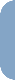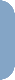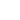> quicklinks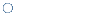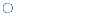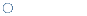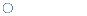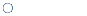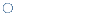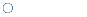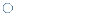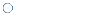quick links ontic tie ultrapascal o> how-to's > how to access a structure

Do you want to include a highscore into your program? No problem, because A68K can do the work for us! How?, we'll explain it here!

>> What is a structure

A structure is a collection of variables, a logical block! For example, a high score can be a structure. It contains a name and the number of points, so how does this structure look? We suggest you to use the following type: {structurename}.{variable/membername} equ {structureposition}
For example our highscore would look like this

 ```highscore.pname equ 0 ; Pointer to the name highscore.wscore equ 4 ; Word, Number of points sizeof_highscore equ 6 ; Size of the highscore structure ```

To calculate the position of your structure, the first index is zero, the next will be the size of the first index, the third [size of first index + size of the second index], ... The last name (sizeof_) should be the size of the structure
Example of a bigger structure

 ```lemming.x_position equ 0 ; X Position, Word lemming.y_position equ 2 ; Y Position, Word lemming.work equ 4 ; Current work, Word lemming.subroutine equ 6 ; Lemming function, Pointer lemming.time equ 10 ; counter, Word sizeof_lemming equ 12 ```

>> access of a structure in assembler

Only problem is the access. You should use pointers to a structure (addressregister), because then you can simply add the value of the position to it. It sounds difficult, but please look at the following example:

 ```; Simple access example lemming.x_position equ 0 ; X Position, Word lemming.y_position equ 2 ; Y Position, Word lemming.work equ 4 ; Current work, Word lemming.subroutine equ 6 ; Lemming function, Pointer lemming.time equ 10 ; counter, Word sizeof_lemming equ 12 ; ProcessLemming ; Input a0 pointer ProcessLemming: move.w lemming.x_position(a0),d0 ; Load x position move.w lemming.y_position(a0),d1 ; Load y position move.l lemming.subroutine(a0),a1 ; Pointer to the routine jsr (a1) ; Call the routine rts _main: lea LemmingStructure(PC),a0 bsr ProcessLemming rts ; Space for 5 lemmings! LemmingStructure ds.b 5*sizeof_lemming ```

And that is all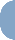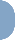powered by ticalc.org, levante.de, xoom.com, listbot.com, fastcounter.com
last update on 12.12.1999